Get instant live expert help with Excel or Google Sheets“My Excelchat expert helped me in less than 20 minutes, saving me what would have been 5 hours of work!”

#### Post your problem and you'll get expert help in seconds

Your message must be at least 40 characters
Our professional experts are available now. Your privacy is guaranteed.

# Learn How to Count Occurrences in an Entire Workbook

We can count occurrences for the number of times a particular value (number, text, date, etc.) occurs across several worksheets in an excel workbook. We can do this by applying the COUNTIF, INDIRECT and SUMPRODUCT functions. We will walk through counting all occurrences in a workbook.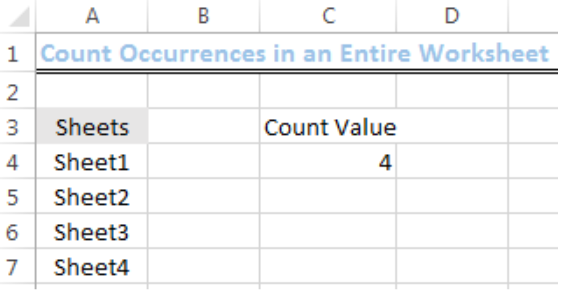Figure 1: Number of Count Occurrences of the number 2 in the workbook

## Naming the Worksheets

We will name the worksheets by doing the following:

• We will rename the worksheet where we want to display our answer as PRIMARY SHEET by right-clicking on it and then, click on RENAME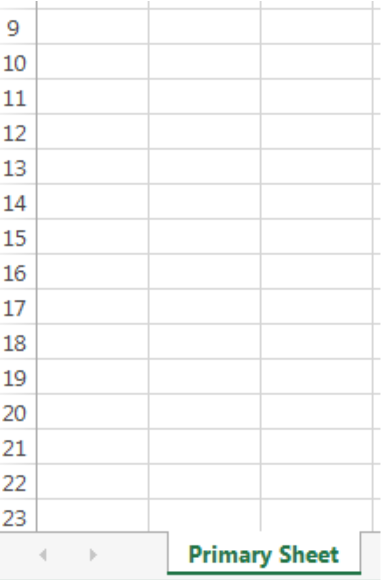Figure 2: Naming the Primary Sheet

• We will create four new worksheets by clicking on the plus sign enclosed in a circle at the bottom of the worksheet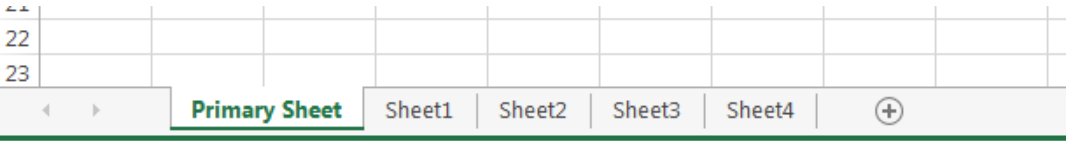Figure 3: Creating Four Sheets

## Setting up the Data

• Our values will be inputted into SHEET1 to SHEET4
• We will input the values as shown in figure 4 into Cell A4 to Cell A8 and name it VALUES with Cell A3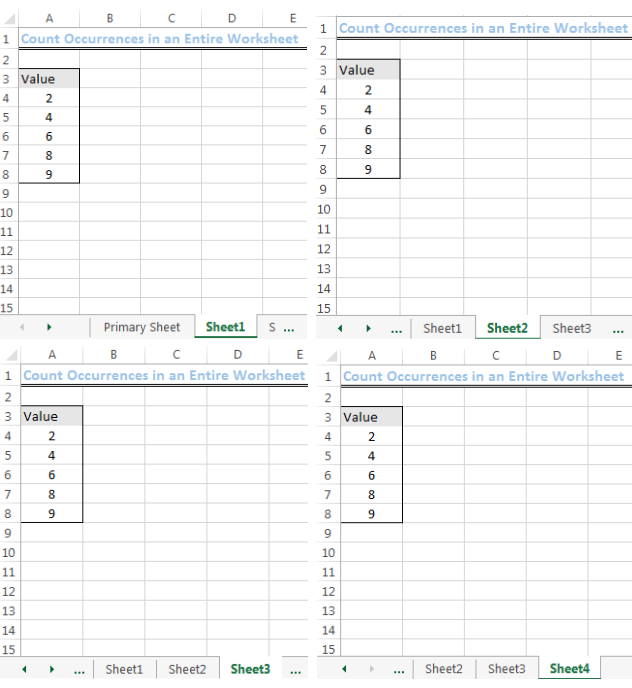Figure 4: Inputting the Values

## Naming the Range of Sheets

• On the Primary sheet, we will input the names of the four worksheets (Sheet1, Sheet2, Sheet3, Sheet4) into Cell A4 to Cell A7
• We will select the range (A4:A7)
• After selecting the range, we will click on the drop-down arrow along the formula bar (fx) and name this range as Sheets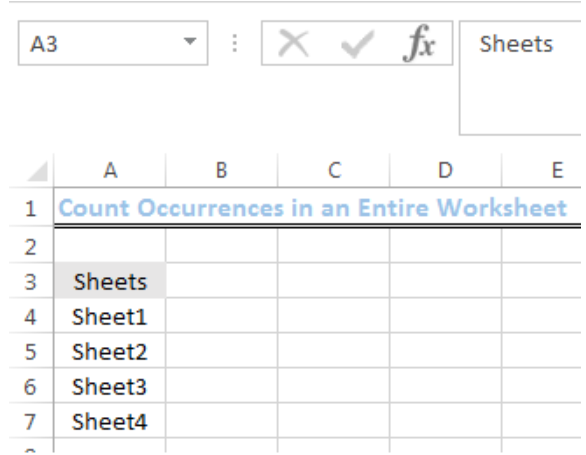Figure 5: Naming the Range

## Syntax to Count Occurrence in a Workbook

`=SUMPRODUCT(COUNTIF(INDIRECT("'"&Sheets&"'!range"),count_value))`

• To count occurrences for this example, we will use the formula below:

`=SUMPRODUCT(COUNTIF(INDIRECT("'"&Sheets&"'!A4:A8"),2))`

## Counting the Number of Occurrences of the Number 2

We will name Cell C3 on the PRIMARY SHEET as Count Value. We will then type or copy and paste the formula into Cell C4. After we have done this, we will press ENTER on the keyboard.

Formula: `=SUMPRODUCT(COUNTIF(INDIRECT("'"&Sheets&"'!A4:A8"),2))`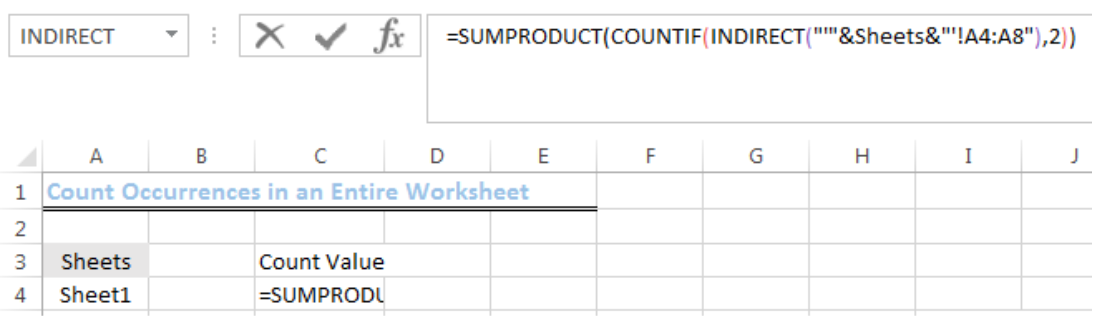Figure 6: Counting Occurrences of the Number 2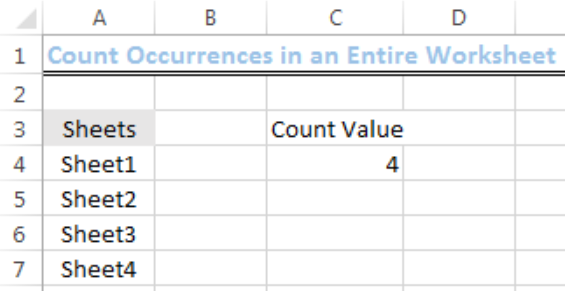Figure 7: Result of the Counted Occurrences of the Number 2

## Explanation of the Formula

The INDIRECT function refers the ranges in the four sheets and the COUNTIF function counts the number of times the value in those ranges match the count value, which is 2. The SUMPRODUCT function then sums the returned count values from each sheet.

## Instant Connection to an Expert through our Excelchat Service

Most of the time, the problem you will need to solve will be more complex than a simple application of a formula or function. If you want to save hours of research and frustration, try our live Excelchat service! Our Excel Experts are available 24/7 to answer any Excel question you may have. We guarantee a connection within 30 seconds and a customized solution within 20 minutes

### Did this post not answer your question? Get a solution from connecting with the expert.Another blog reader asked this question today on Excelchat:## Subscribe to Excelchat.coAnother blog reader asked this question today on Excelchat: